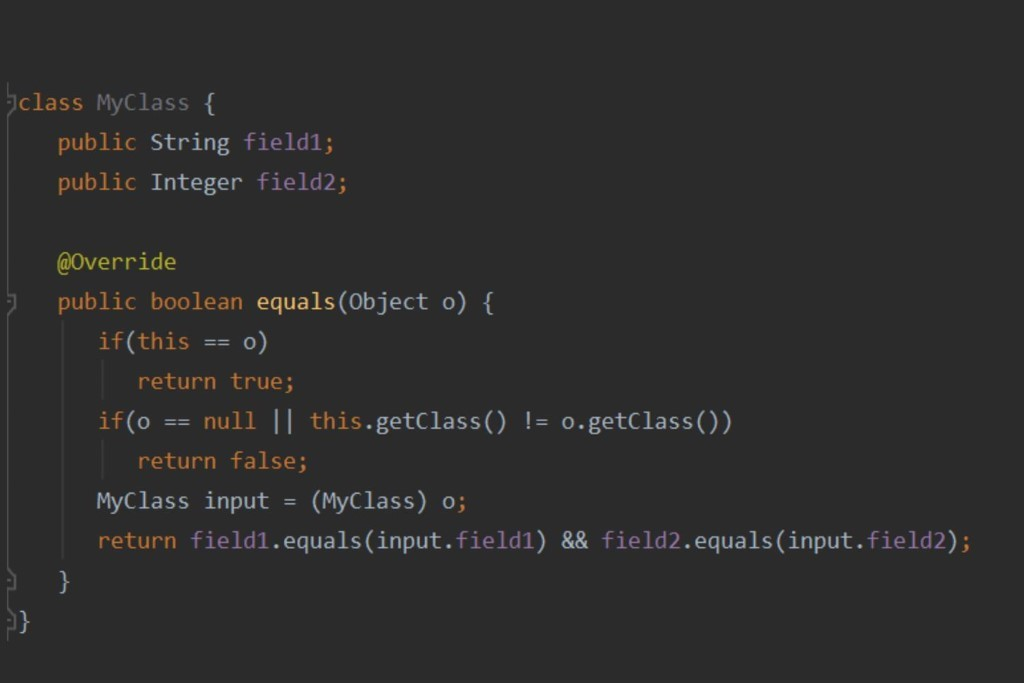19849
18Hard skills
Learning Java. equals() and hashCode() Methods
21 Jul 2020

In this article, we will take a look at two important methods of the Object class in Java, equals() and hashCode(), find out what is their purpose and how and when to use them, with concrete examples.

### What is the purpose of equals() and hashCode() and what features they have?

Comparing objects is a pretty common situation in programming. The first thing that comes to mind when you want to compare something is to check the condition with the “==” symbol. It seems like a solution to all our problems, only it is not. It makes sense to use the "==" symbol with primitives though. As for objects, in Java "==" compares references, that is, it checks if the object is equal to itself, which may not always be useful. In the Object class, there is an equals() method which is actually intended for object comparison. By default, the equals() method performs the "==" operation internally, however, the idea behind this method is that it must be overridden for the created classes.

### What should the overridden equals() method look like?

The method looks like this: public boolean equals (Object o);

It accepts Object as input and should return true or false. Now let's go over the steps.

### How should the comparison of two objects in Java be performed for it to be efficient?

1) When comparing two objects, it stands to reason starting with reference check. After all, if we are comparing an object to itself, why do we need to perform unnecessary checks and compare the object's fields.

2) Before proceeding to in-depth comparison, we need to perform 2 more checks, which can also filter out objects that are definitely not equal. This will speed up the operation of the equals() method. These checks help us figure whether the object is null and whether the compared objects are of the same classes. If we initially compare two null objects, then the first check will return true, and by the second step we will be left with the objects that are most certainly not null.

3) Now that we are sure that both objects belong to the same class and are not null, we can cast the object to the desired type and start comparing the fields.

#### Example

And here is an example of the overridden method we have got:Not bad, but take note that the equals() methods invoked for String and Integer are already overridden within these classes. If the field of your class is an object of your other class, it is also necessary to override the equals() method in it. Moreover, the presented method fully complies with the specific requirements, spelled out in the Oracle documentation:

1) It is reflexive. Any non-null object must be equals() to itself.

2) It is symmetric. If a.equals (b) == true, then b.equals (a) must return true.

3) It is transitive. If two objects are equal to some third object, then they must be equal as well.

4) It is consistent. The results of the equals() method should only change when its input fields are modified. If the data of two objects has not changed, the results of the equals() test should always be the same.

5) Inequality with null. For any non-null object, the check a.equals (null) must return false.

### But what if we just need to make sure that the objects are not equal and do so very quickly?

This approach is used when we need to store a set of unique objects, for example, in such data structure as HashMap. In this case, the equals() method is not very suitable, since it takes time to check all fields of the object. The hash code of the object is more fitting for the purpose.

In Java, the object’s hashcode can be obtained using the hashCode() method. For any object, this method returns a 32-bit value of the int type. The returned hash code must meet the following requirements specified in the Oracle documentation:

1) If two objects are equal (i.e. the equals() method returns true), they must have the same hashcode.

2) If the hashCode() method is called multiple times on the same object, it must return the same result every time.

3) Two different objects can have the same hash code.

The last rule stems from the fact that the hash code is limited to 32 bits, that is, it can take a little more than 4 billion different values, while the number of objects created is not limited by anything except the memory available to the application.

#### Example

Let's consider how the hashCode() method can be overridden using our class as an example:Multiplication by a prime odd number 31 is used to reduce the number of collisions, that is, to make the variety of calculated hash codes as large as possible. There is also a more visually pleasing way to override the hash code. The Objects class has a hash() method, to the input of which all the fields of the class are fed. This method does the same thing as the one, shown in the previous picture:Using a hashcode allows you to efficiently manipulate elements in a HashMap. How exactly this efficiency of HashMap is achieved, what problems it solves and what problems you may encounter when working with it, we will share in one of the following articles.